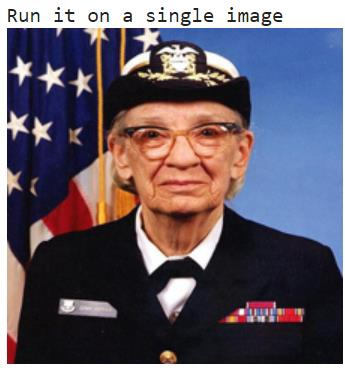# How can Tensorflow be used to download a single image to try the model on using Python?

TensorflowServer Side ProgrammingProgrammingPython

Tensorflow can be used to download a single image to try the model using the ‘get_file’ method. A google API holds the single image, which can be passed as parameter to the ‘get_file’ method to download the dataset in the current environment.

A neural network that contains at least one layer is known as a convolutional layer. We can use the Convolutional Neural Network to build learning model.

We are using the Google Colaboratory to run the below code. Google Colab or Colaboratory helps run Python code over the browser and requires zero configuration and free access to GPUs (Graphical Processing Units). Colaboratory has been built on top of Jupyter Notebook.

The intuition behind transfer learning for image classification is, if a model is trained on a large and general dataset, this model can be used to effectively serve as a generic model for the visual world. It would have learned the feature maps, which means the user won’t have to start from scratch by training a large model on a large dataset.

TensorFlow Hub is a repository that contains pre-trained TensorFlow models. TensorFlow can be used to fine-tune learning models.

We will understand how to use models from TensorFlow Hub with tf.keras, use an image classification model from TensorFlow Hub.  Once this is done, transfer learning can be performed to fine-tune a model for customized image classes. This is done by using a pretrained classifier model to take an image and predict what it is. This can be done without needing any training.

## Example

print("Run it on a single image")
grace_hopper = Image.open(grace_hopper).resize(IMAGE_SHAPE)
grace_hopper

## Output

Run it on a single image
65536/61306 [================================] - 0s 0us/step